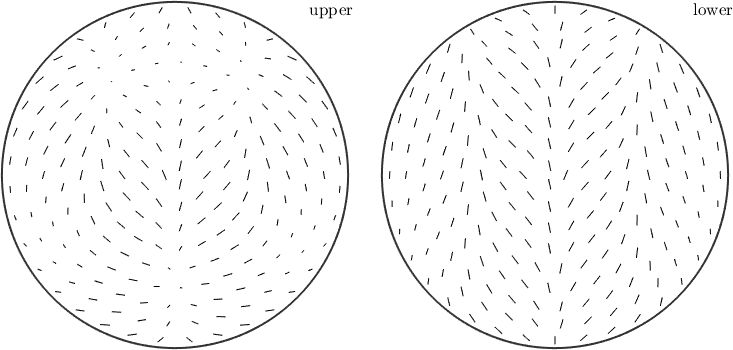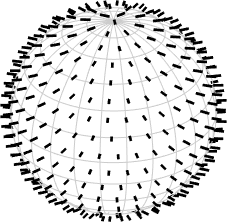S2AxisFieldHarmonic edit page

S2AxisFieldharmonic handles axis fields on the sphere. Axis can be understood as three-dimensional vectors without direction or length.

S2AxisFieldHarmonic handles functions of the form

$f\colon {\bf S}^2\to{\bf R}^3_{/<\pm \mathrm{Id}>}.$

## Defining a S2AxisFieldHarmonic

Definition via function values

At first you need some vertices

Next you define function values for the vertices

Now the actual command to get sAF1 of type S2AxisFieldHarmonic

Definition via function handle

If you have a function handle for the function you could create a S2AxisFieldHarmonic via quadrature. At first lets define a function handle which takes vector3d as an argument and returns antipodal vector3d:

Now you can call the quadrature command to get sAF2 of type S2AxisFieldHarmonic

## Visualization

One can use the default plot-command• same as quiver(sAF1)

or use the 3D plot of a sphere with the axis on itself